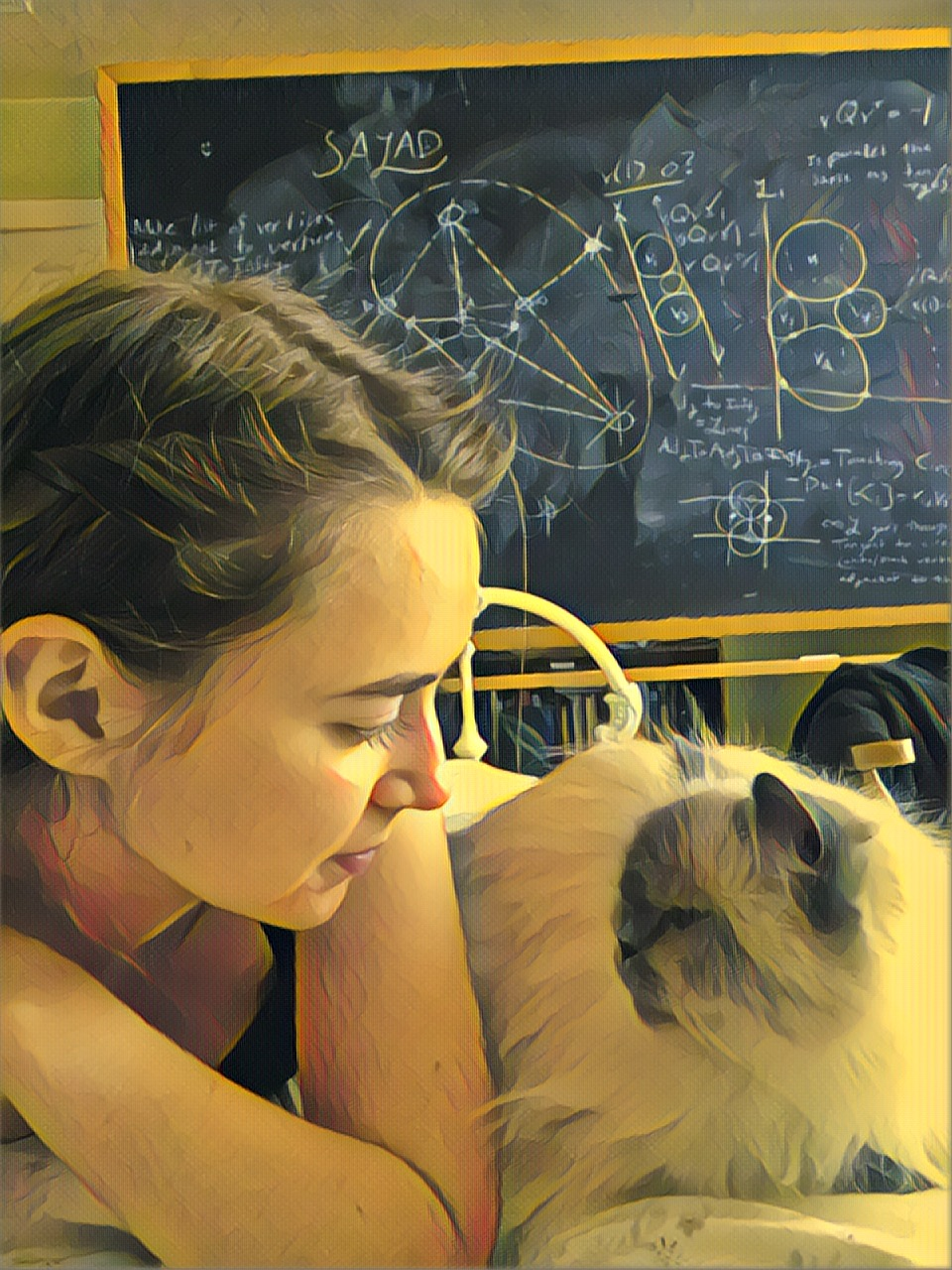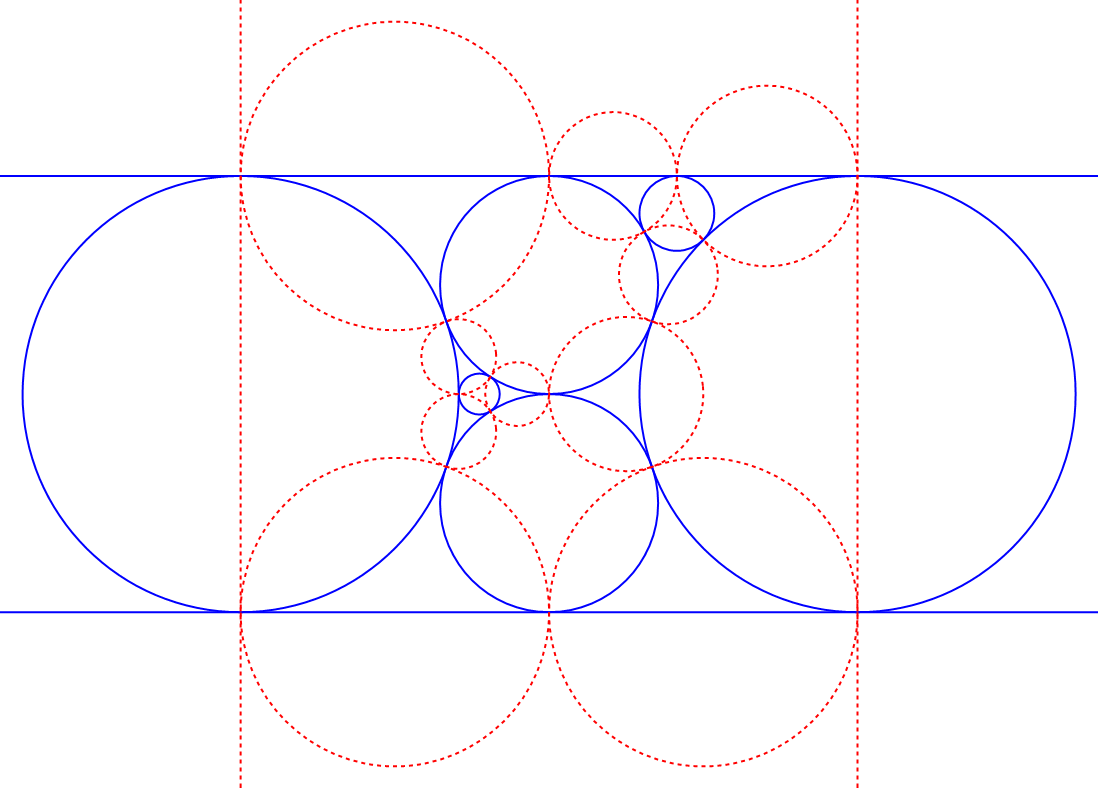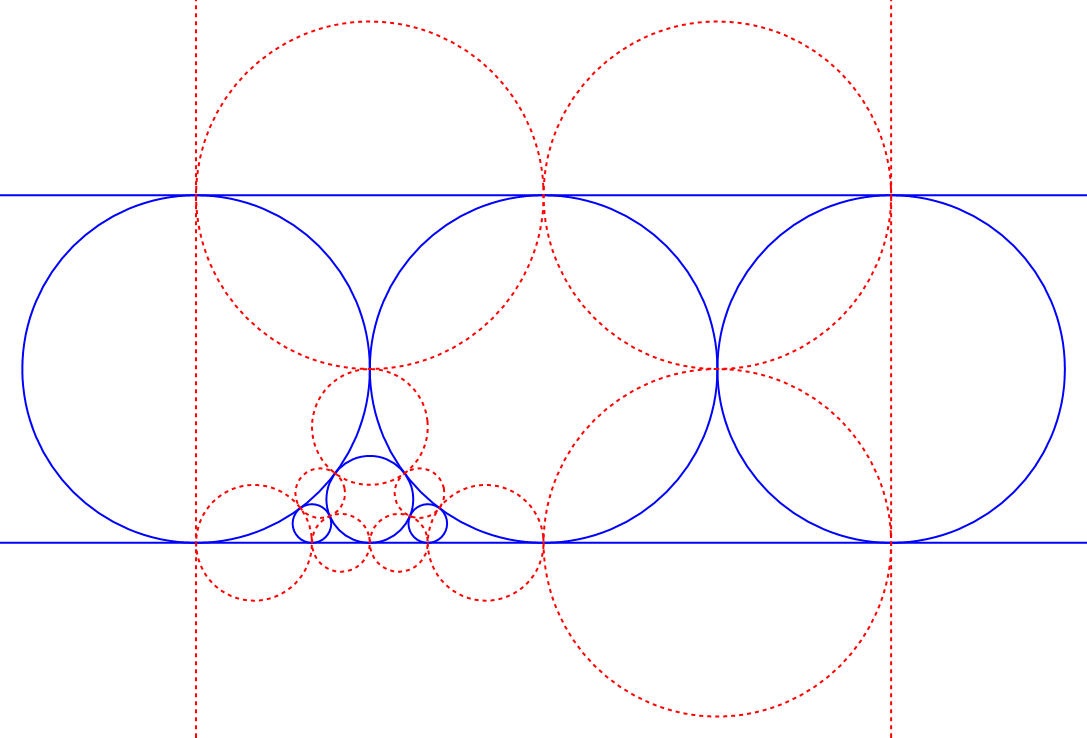# Brittany Gelb's REU 2020 Web PageName: Email: Brittany Gelb begelb (at) muhlenberg.edu Muhlenberg College Number Theory and Hyperbolic Geometry Professor Alex Kontorovich

## About My Project: Classifying Integral Polyhedra

The Koebe-Andreev-Thurston Theorem implies that there exists a mapping from every convex polyhedron* to a cluster of circles, a finite arrangement of mutually tangent or disjoint circles. Such a cluster can be extended to an infinite packing of circles by means of the Structure Theorem for Crystallographic Packings (Kontorovich, Nakamura 2018). For example, the packing that arises from a tetrahedron is a classical structure known as the Apollonian Gasket. We define the bend of a circle to be the reciprocal of the radius. A packing such that all circles have integer bend is an integral packing. If a polyhedron gives rise to an integral packing, it is an integral polyhedron. Kontorovich and Nakamura (2018) have shown that there exist infinitely many integral polyhedra. The objective of my work is to provide a complete classification of integral polyhedra using the framework developed by Kontorovich and Nakamura. For example, their works suggests that it may be possible to identify a finite list of “seed” polyhedra from which all integral polyhedra can be obtained.

This work is supported by supported by the Rutgers Department of Mathematics and the National Science Foundation Grant DMS-1802119.

For other exciting work in number theory and hyperbolic geometry, see the websites of my colleagues: Colin Fan, Saket Shah, Kai Shaikh, and Brandon Gomes.

*Throughout this website, I will always be referring to convex polyhedra when I write polyhedra.

## Research Log

### Week 1: May 26 - May 31

The goal of this week was to gain an introductory understanding of the research topics through lectures by Professor Kontorovich and guest lectures by Professor Jeremy Kahn. By Friday, each member of the group narrowed down their interests to a topic to pursue. I'm interested in how hyperbolic geometry and number theory will become tools for the problem I describe above, which on the surface seems comfortably seated in Euclidean geometry. I've started to read Letter to Bill Duke on "Inversive Coordinates," which discusses a coordinate system for the upper half plane model of hyperbolic geometry. In addition, I've started working to understand the proof of the Koebe-Andreev-Thurston theorem as exposited in Convex Polytopes: Extremal Constructions and f-Vector Shapes by G. Ziegler. Finally, I've started to gain a broad overview of the work that has already been done on this question in Geometry and arithmetic of crystallographic sphere packings by Kontorovich and Nakamura.

### Week 2: June 1 - June 7

This week I started a computational investigation of integral polyhedra. I also gave a talk introducing the topic of my research. My near-future goal is to write an algorithm following the proof of the Koebe-Andreev-Thurston Theorem to map a polyhedron to its associated circle packing. A useful theorem by Steinitz states that there is a bijection between 3-connected planar graphs and combinatorial types of polyhedra.

A non-important explanation of what I mean when I use the phrase combinatorial types of polyhedra: In the context of polyhedra, a face is a general term that includes facets, edges, and vertices. We say that two polyhedra are of the same combinatorial type if there exists a one-to-one correspondence between their faces so that two faces of one polyehdron meet if and only if the two corresponding faces of the other polyhedron meet. For my non-technical audience, you can think of two polyhedra as being of the same combinatorial type if they have the same number of each type of face and you can get from one to the other by only stretching faces while preserving convexity, i.e. not causing dents to appear.

Due to the theorem by Steinitz, I can use 3-connected planar graphs at the input for my algorithm. This week I familiarized myself with the program plantri as a way of generating all 3-connected planar graphs, which will serve as the input to my program. My progress so far is code that computes the overlay of a graph and its dual.

### Week 3: June 8 - June 14

This week I continued to work on the algorithm. After computing the overlay graph, I can now compute what is called a restricted quad graph and then optimize some properties about it so that it becomes easy to transform it into a circle packing. I ran into some problems using the built in Sage function "minimize with constraints," so I wrote a gradient descent function to solve the optimization problem. This is the restricted quad graph for the cube, which happened to look like a sting ray.

### Week 4: June 15 - June 21

This week I wrote code to compute the radii and Cartesian coordinate centers for each circle in the circle cluster. After seeing that my previous method failed for certain examples, I also developed a way to solve the optimization problem that is more robust.

### Week 5: June 22 - June 28

I'm now able to compute the inversive coordinates for each generalized circle of the circle cluster. From this I can compute the Gram matrix, which contains all of the important data about a circle cluster you could obtain from the geometric rendering: Which circles are tangent and which circles intersect orthogonally? If two circles are disjoint, what is the distance between them?

### Week 6: June 29 - July 6

The Gram matrix for a circle packing is known to have all algebraic entries. This week I wrote code to find nearby algebraic numbers based on numerical approximations to those numbers. I made partial progress towards increasing the overall precision of my algorithm to improve the precision of the final result.

### Week 7: July 6 - July 12

This week I translated my code from Sage to Mathematica due to a variety of technical problems with precision in the computations. I was able to look at several polyhedra with 8 vertices and generate the associated circle cluster data. The second and third pictures in the slideshow feature circle clusters that give rise to integral packings. The cluster shown with solid, blue lines is generated from one polyhedron. The co-cluster is shown with dotted, red lines, and it is generated from the dual polyhedron. Notice that whenever two circles from the cluster and co-cluster intersect, they intersect orthogonally. Also note that the straight lines shown in the pictures are circles of infinite radii.
1 / 3Caption Text
2 / 3Caption Two
3 / 38v12f_7

### Week 8: July 13 - July 18

This week I automated the code so that it could generate large amounts of data. I learned techniques for proving the integrality of circle packings and discovered 15 new integral polyhedra on 8 and 9 vertices. It is computationally expensive but not impossible to investigate all polyhedra on 9 vertices.

### Week 9: July 19 - July 24

This week was spent preparing for the final presentation and writing the final report. The Appendix to my NSF report is here. You can find my code on Github.

## Polyhedral Circle Packings

Polyhedron Strip Supercluster Strip Packing Gramian Matrix Inversive Coordinates Bend Matrices Mathematica File
TetrahedronIntegral
Code